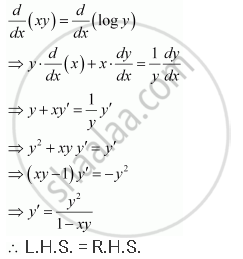Share

# Verify that the Given Functions (Explicit Or Implicit) is a Solution of the Corresponding Differential Equation Xy = Log Y + C : Y' = (Y^2)/(1 - Xy) (Xy != 1) - Mathematics

#### Question

verify that the given functions (explicit or implicit) is a solution of the corresponding differential equation

xy = log y + C :  y' = (y^2)/(1 - xy) (xy != 1)

#### Solution

xy = log y + C

Differentiating both sides of this equation with respect to x, we get:Hence, the given function is the solution of the corresponding differential equation.

Is there an error in this question or solution?
Verify that the Given Functions (Explicit Or Implicit) is a Solution of the Corresponding Differential Equation Xy = Log Y + C : Y' = (Y^2)/(1 - Xy) (Xy != 1) Concept: General and Particular Solutions of a Differential Equation.